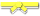# Search Results

Searching for: bag

## Individual Exercises

0 / 50

Write a member method balanceBags in Java that implements the following logic: Given a String parameter the methods returns the String...0 / 50

Write the member method 'playBagBingo()' that implements the player logic for the game described below. Bag Bingo is a game of chance...0 / 30

For this assignment, create a method that will turn a fixed-size arrayBag into a set.

A Set is an abstract data type somewhat similar...0 / 50

Write the toString member method that returns the String representation of the items in the bag in the form [item, item, item, item]. For...0 / 50

delete this question0 / 50

For the question below, assume the following implementation of the Ball class

``public class Ball{ private String color; private double...``0 / 50

For this question, assume the following implementation of the class Person and the following UML diagram

#### Person Class Implementation

``...``0 / 50

For this question, assume the following implementation of the class Person and the following UML diagram

#### Person Class Implementation

``...``0 / 50

Blank diagram.png0 / 50

For the question below, assume the following implementation of the Measurable interface and Square and Circle classes:

``public interface ...``0 / 50

For this question assume the following implementations of Computer, Tablet, and Notebook

``public class Computer { private String...``0 / 50

For this question, assume the following implementation of the Person and Student classes:

#### Person Class Implementation

``public class Person...``0 / 50

For the question below, assume the following implementation of the Measurable interface and Square and Circle classes:

``public class Shape...``0 / 50

For this question assume the following implementations of Computer, Tablet, and Notebook

``public class Computer { private String...``0 / 50

For this question assume the following implementations of Computer, Tablet, and Notebook

``public class Computer { private String...``0 / 50

For this question, assume the following implementation of the Person and Student classes:

#### Person Class Implementation

``public class Person...``0 / 50

For the question below, assume the following implementation of the Measurable interface and Square and Circle classes:

``public class Shape...``0 / 50

Write a `LinkedBag<T>` member method called `contains()` that takes an element as a parameter and returns true if the bag contains the...0 / 50

For this exercise, you are writing code in the following implementation of ArrayBag:

``public static final class ArrayBag<T> ...``0 / 50

For this exercise, you are writing code in the following implementation of ArrayBag:

``public static final class ArrayBag<T> ...``0 / 50

For this question you will be writing a method `reverse` that takes in two parameters.

``public T[] reverse(T[] arr, T[] reversedArr) ``

The...0 / 50

For this question you will be writing a method `reverse` that takes in two parameters.

``public T[] truncate(T[] arr, T[] truncatedArr) ``

The...0 / 50

For this question, you will be given all the code necessary except the parameters. This method needs to take in a List of any type of...0 / 50

For this question, you will need to both write the method and add in the correct parameter.

This method needs to take in a List of any...0 / 50

For this question assume the following implementations of Computer, Tablet, and Notebook

``public class Computer { private String...``